It’s fair to say that using functions is the biggest advantage of Python. Or at least you will use them a lot during your Data Science projects! This is episode #3 of the “Python for Data Science Basics” series and it’s about the Python functions and methods!

In this article I won’t just introduce you to the concept, but will give you a list of the most important functions and methods that you will use all the time in the future.

Note: As always, this is a hands-on tutorial. I highly recommend doing the coding part with me – and if you have time, solve the exercises at the end of the article! If you haven’t done so yet, please go through these three articles first:

## What are Python functions and methods?

`a = 'Hello!'`

Here’s a simple example of a Python function:
`len(a)`
Result: `6`

And an example for a Python method:
`a.upper()`
Result: `'HELLO!'`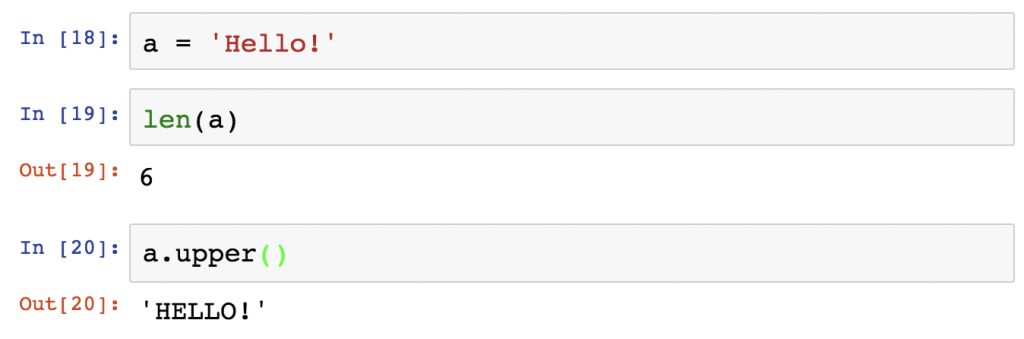So what are Python functions and methods? In essence they transform something into something else. In this case the input was `'Hello!'` and the output was the length of this string (6), and then the capitalized version: `'HELLO!'`. Of course, these are not the only 2 functions you can use: there are plenty of them. Combining them will help you in every part of your data project – from data cleaning to machine learning. Everything.

## Built-in vs. user-defined functions and methods

The cool thing is that besides the long list of built-in functions/methods, you can create your own too! Also, you will see that when you download, installing and importing different Python libraries, they will come with extra functions and methods as well. So there are indeed infinite possibilities. I’ll get back to this topic later. For now, let’s focus on the built-in things.

## The most important built-in Python functions for data projects

Python functions work very simply. You call the function and specify the required arguments, then it will return the results. The type of the argument (e.g. string, list, integer, boolean, etc…) can be restricted (e.g. in some cases it has to be an integer), but in most cases it can be multiple value types. Let’s take a look at the most important built-in Python functions:

`print()`
We have already used `print()`. It prints your stuff to the screen.
Example: `print("Hello, World!")`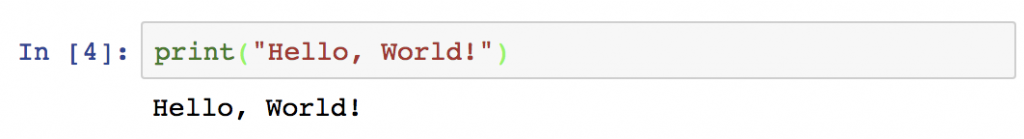`abs()`
returns the absolute value of a numeric value (e.g. integer or float). Obviously it can’t be a string. It has to be a numeric value.
Example: `abs(-4/3)`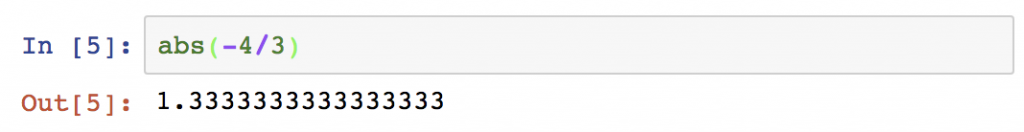`round()`
returns the rounded value of a numeric value.
Example: `round(-4/3)`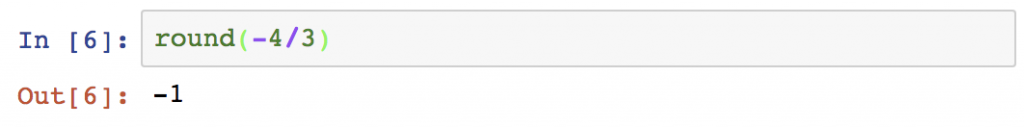`min()`
returns the smallest item of a list or of the typed-in arguments. It can even be a string.
Example 1: `min(3,2,5)`
Example 2: `min('c','a','b')`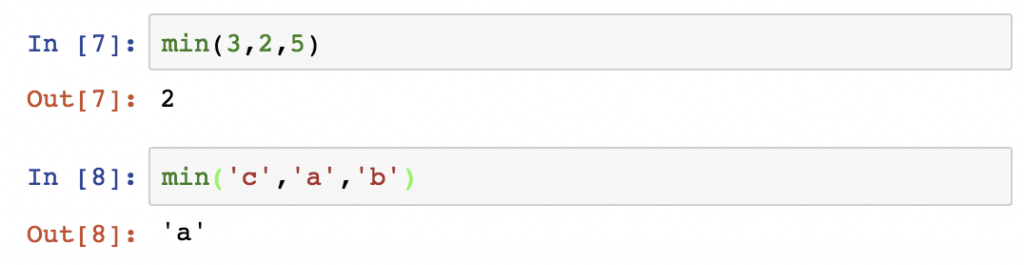`max()`
Guess, what! It’s the opposite of `min()`. 🙂

`sorted()`
It sorts a list into ascending order. The list can contain strings or numbers.
Example:
`a = [3, 2, 1]`
`sorted(a)`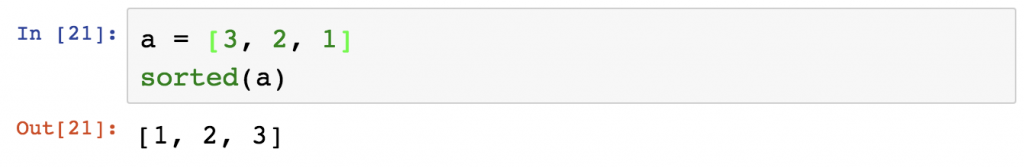`sum()`
It sums a list. The list can have all types of numeric values, although it handles floats… well, not smartly.
Example1:
`a = [3, 2, 1]`
`sum(a)`
Example1:
`b = [4/3, 2/3, 1/3, 1/3, 1/3]`
`sum(b)`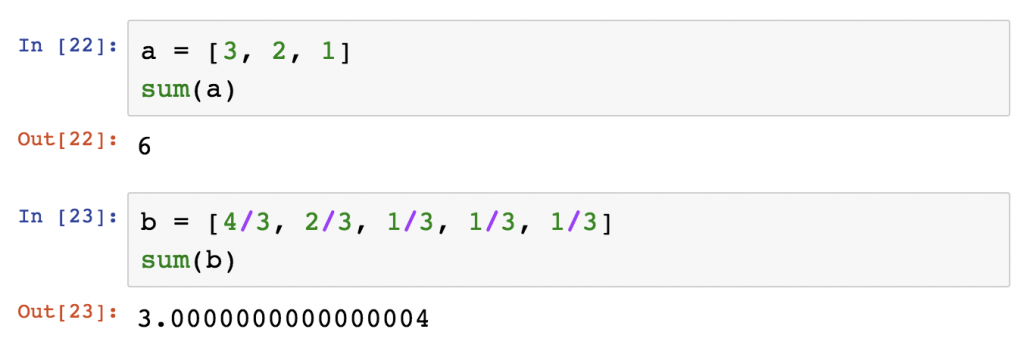`len()`
returns the number of elements in a list or the number of characters in a string.
Example: `len('Hello!')`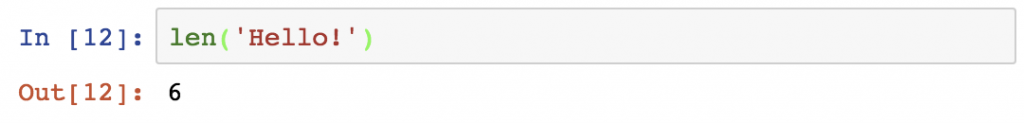`type()`
returns the type of the variable.
Example 1:
`a = True`
`type(a)`
Example 2:
`b = 2`
`type(b)`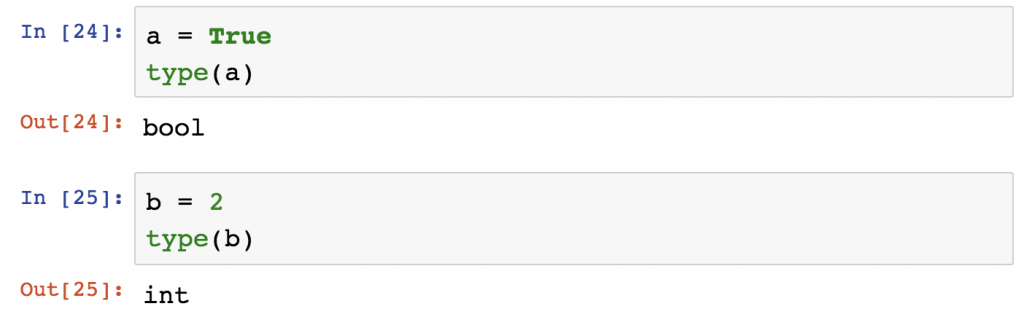These are the built-in Python functions that you will use quite regularly. If you want to see all of them, here’s the full list: https://docs.python.org/3/library/functions.html

But I’ll also show you more in my upcoming tutorials.

## The most important built-in Python methods

Most of the Python methods are applicable only for a given value type. Eg. `.upper()` works with strings, but doesn’t work with integers. And `.append()` works with lists only and doesn’t work with strings, integers or booleans. So I’ll break down the methods by value type!

## Methods for Python Strings

The string methods are usually used during the data cleaning phase of the data project. E.g. imagine that you collect data about what people are searching for on your ecommerce website. And you find these strings: `'mug'`, `'mug '`, `'Mug'`. You know that this is the same, but to let Python know too, you should handle this situation! Let’s see the most important string methods in Python:

`a.lower()`
returns the lowercase version of a string.
Example:
`a = 'MuG'`
`a.lower()`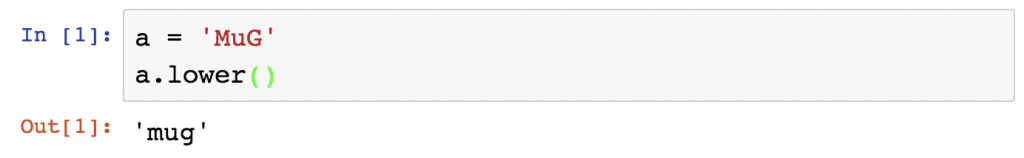`a.upper()`
the opposite of `lower()`

`a.strip()`
if the string has whitespaces at the beginning or at the end, it removes them.
Example:
`a = ' Mug '`
`a.strip()`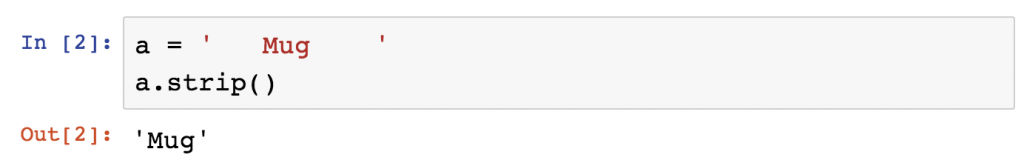`a.replace('old', 'new')`
replaces a given string with another string. Note that it’s case sensitive.
Example:
`a = 'muh'`
`a.replace('h','g')`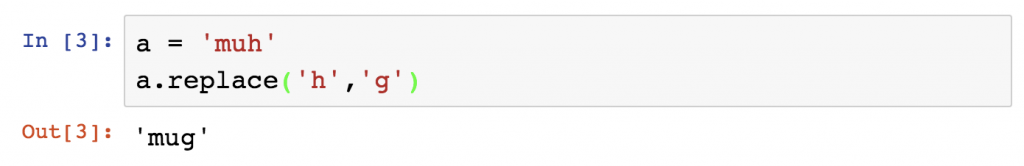`a.split('delimiter')`
Example:
`a = 'Hello World'`
`a.split(' ')`
Note: in this case the space is the delimiter.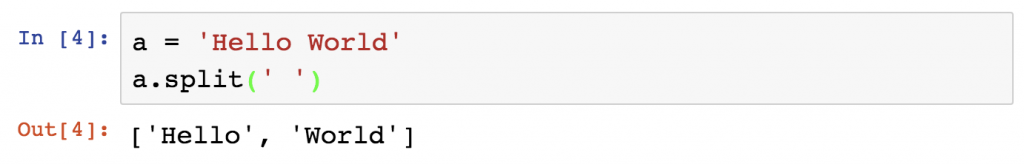`'delimiter'.join(a)`
It joins elements of a list into one string. You can specify the delimiter again.
Example:
`a = ['Hello', 'World']`
`' '.join(a)`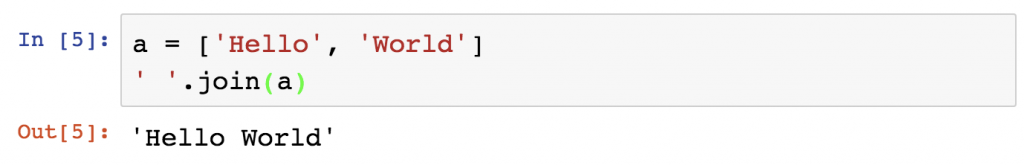Okay, that’s it for the most important string methods in Python.

## Methods for Python Lists

Do you remember the last article, when we went through the Python data structures? Let’s talk a little bit about them again. Last time we discussed how to create a list and how to access its elements. But I haven’t told you about how to modify a list. Any tips? Yes, you will need the Python List methods!

Let’s bring back our favorite Python Dog, Freddie:
`dog = ['Freddie', 9, True, 1.1, 2001, ['bone', 'little ball']]`

Let’s see how we can modify this list!
`a.append(arg)`
The `.append()` method adds an element to the end of our list. In this case, let’s say we want to add the number of legs Freddie has (which is 4).
Example:
`dog.append(4)`
`dog`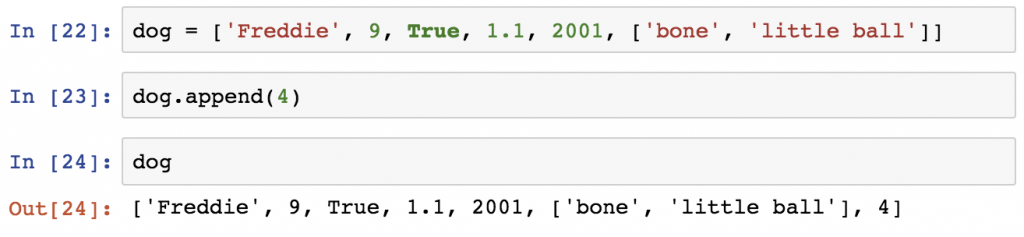`a.remove(arg)`
If we want to remove the birth year, we can do it using the `.remove()` method. We have to specify the element that we want to remove and Python will remove the first item with that value from the list.
`dog.remove(2001)`
`dog`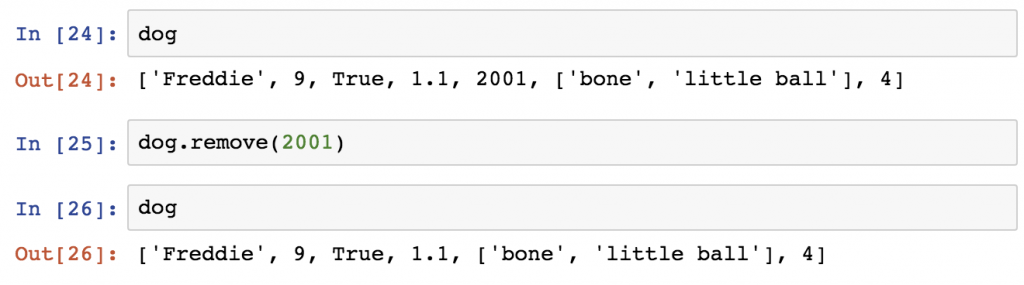`a.count(arg)`
returns the number of the specified value in a list.
Example:
`dog.count('Freddie')`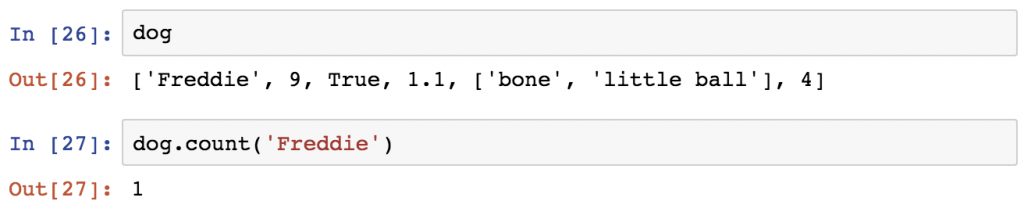`a.clear()`
removes all elements of the list. It will basically delete Freddie. No worries, we will get him back.
Example:
`dog.clear()`
`dog`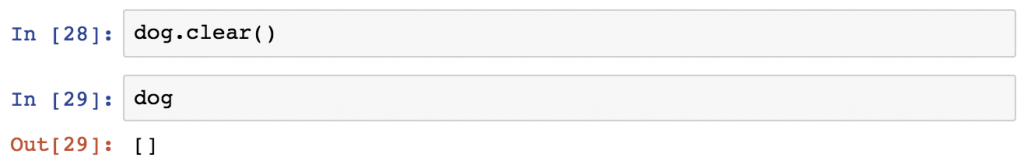By the way, here you can find the full list of list methods in Python: https://docs.python.org/3/tutorial/datastructures.html

## Methods for Python Dictionaries

As with the lists, there are some important dictionary functions to learn about.

Here’s Freddie again (see, I told you he’d be back):

```dog_dict = {'name': 'Freddie',
'age': 9,
'is_vaccinated': True,
'height': 1.1,
'birth_year': 2001,
'belongings': ['bone', 'little ball']}
```

`dog_dict.keys()`
will return all the keys from your dictionary.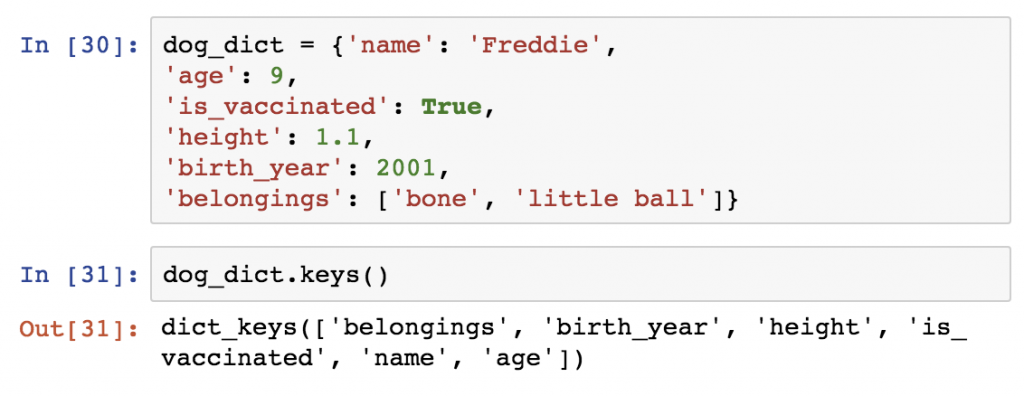`dog_dict.values()`
will return all the values from your dictionary.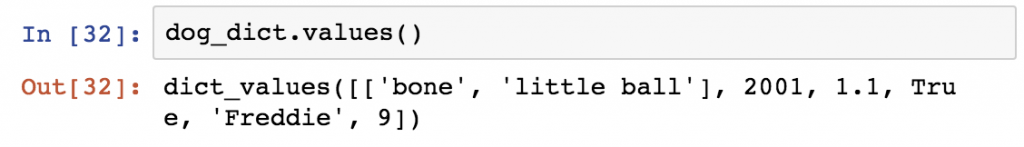`dog_dict.clear()`
will delete everything from your dictionary.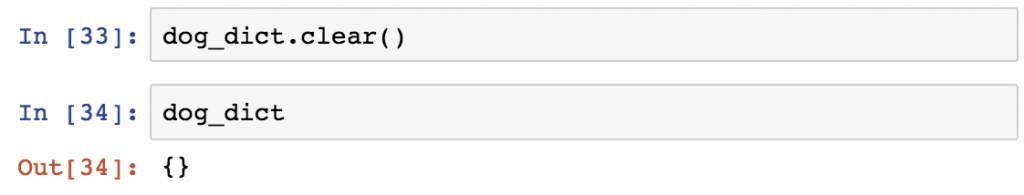Note:
Adding an element to a dictionary doesn’t require you to use a method; you have to do it by simply defining a key-value pair like this:
`dog_dict['key'] = 'value'`
Eg.
`dog_dict['name'] = 'Freddie'`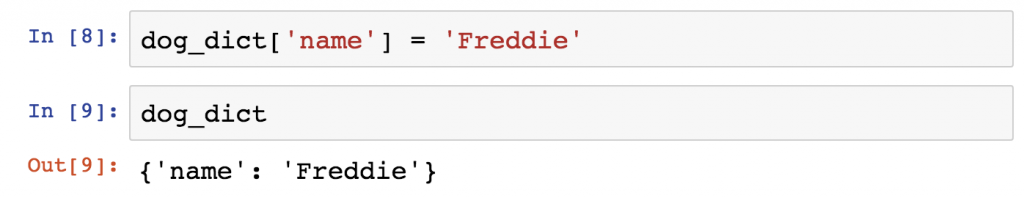Okay, these are all the methods you should know for now! We went through string, list and dictionary Python methods!
It’s time to test yourself!

## Test yourself!

For this exercise you will have to use not only what you have learned today, but what you have learned about Python Data Structures and variable types too! Okay, let’s see:

1. Take this list:
`test_yourself = [1, 1, 2, 2, 3, 3, 3, 3, 4, 5, 5]`
2. Calculate the mean of the list elements – by using only those things that you have read in this and the previous articles!
3. Calculate the median of the list elements – by using only those things that you have read in this and the previous articles!

.

.

.

And the solutions are:
2) `sum(test_yourself) / len(test_yourself)`
Where the `sum()` sums the numbers and the `len()` counts the elements. The division of those will return the mean. The result is: `2.909090`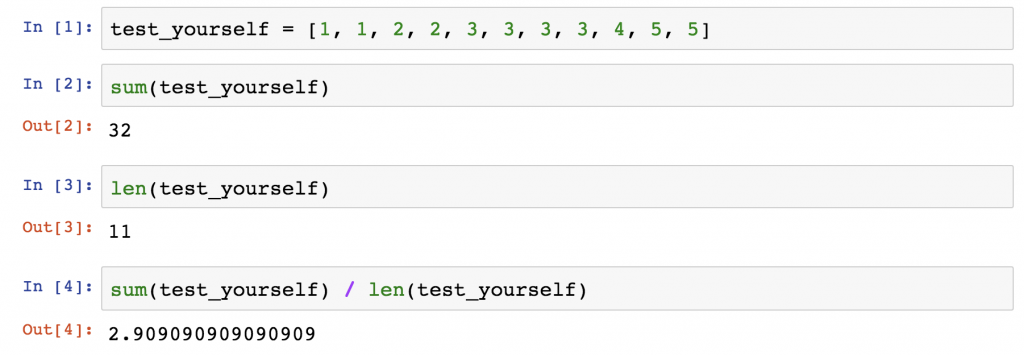3) `test_yourself[round(len(test_yourself) / 2) - 1]`
We are lucky to have a list with an odd number of elements.

Note: this formula won’t work for a list with an even number of elements.

`len(test_yourself) / 2` will basically tell us where in the list we should look for our middle number – which will be the median. The result is `5.5`, but in fact the result of `len() / 2` will always be less by `0.5` than our exact number – when the list has odd number of elements (check it out for a 3 or 5-element list too). So let’s round this `5.5` up to `6` by using `round(len(test_yourself) / 2)`. That’s right: we can put a function into a function. Then subtract one because of the zero-based indexing: `round(len(test_yourself) / 2) - 1`

And eventually use this result as the index of the list: `test_yourself[round(len(test_yourself) / 2) - 1]` or replace it with the exact number: `test_yourself`. The result is: `3`.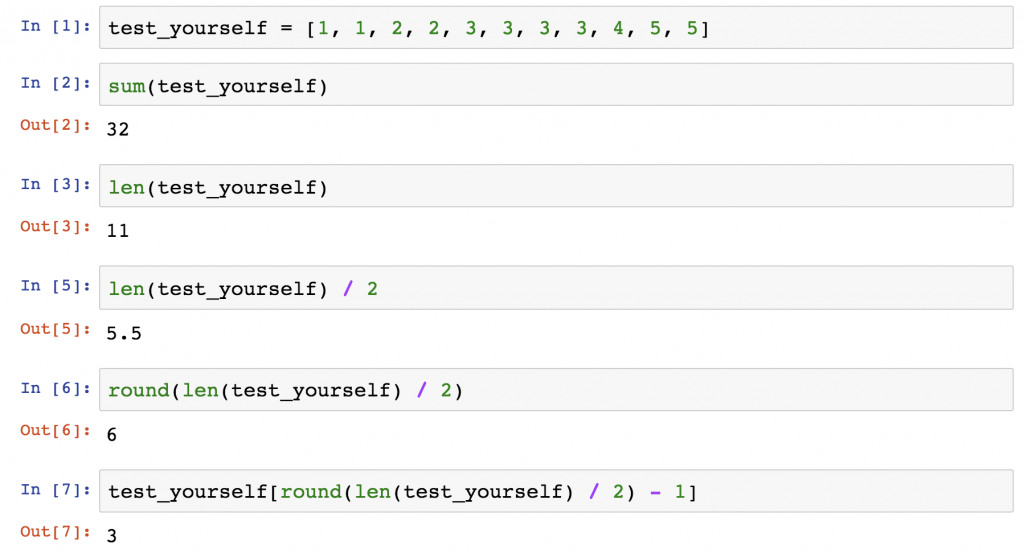## What’s the difference between Python functions and methods?

After reading this far in the article, I bet you have this question: “Why on Earth do we have both functions and methods, when they practically do the same thing?

I remember that when I started learning Python, I had a hard time answering this question. This is still the most confusing topic for newcomers in the Python-world… The full answer is very technical and you are not there yet. But here’s a little help for you to avoid confusion.

Firstly, start with the obvious. There is a clear difference in the syntax:
A function looks like this: `function(something)`
And a method looks like this: `something.method()`
(Look at the examples above!)

So why do we have both methods and functions in Python? The official answer is that there is a small difference between them. Namely: a method always belongs to an object (e.g. in the `dog.append(4)` method `.append()` needed the `dog` object to be applicable), while a function doesn’t necessarily. To make this answer even more twisted: a method is in fact nothing else but a specific function. Got it? All methods are functions, but not all functions are methods!

If this makes no sense to you (yet), don’t you worry. I promise, the idea will grow on you as you use Python more and more – especially when you start to define your own functions and methods.

But just in case, here’s a little extra advice from me:
In the beginning, learning Python functions and methods will be like learning the articles (der, die, das) of the German language. You have to learn the syntax, use it the way you have learned and that’s it.

Just like in German, there are some general rules of thumb that can help you recall things. The main one is that functions are usually applicable for multiple type of objects, while methods are not. E.g. `sorted()` is a function and it works with strings, lists, integers, etc. While `.upper()` is a method and it only works with strings.

But again: my general advice here is that you should not put too much effort into understanding the difference between methods and functions at this point; just learn the ones I mentioned in this article and you’ll be a happy Python user.

## Conclusion

Great, you have learned 20+ Python methods and functions. This is a good start, but remember: these are only the basics. In the next episodes, we will rapidly extend this list by importing new data science Python libraries with new functions and new methods!

As a next step, let’s learn a bit about loops and if statements! Here is the link to continue: Python If Statements (Explained).

Cheers,
Tomi Mester